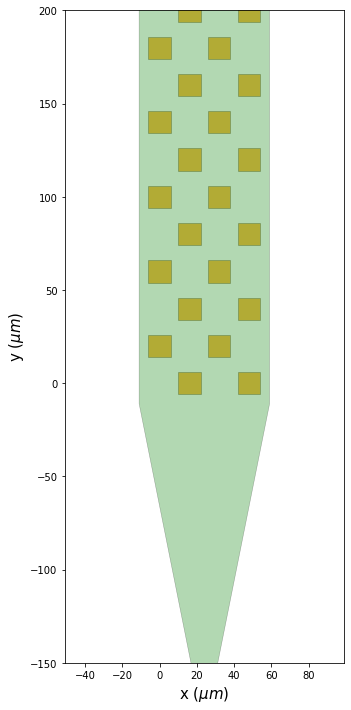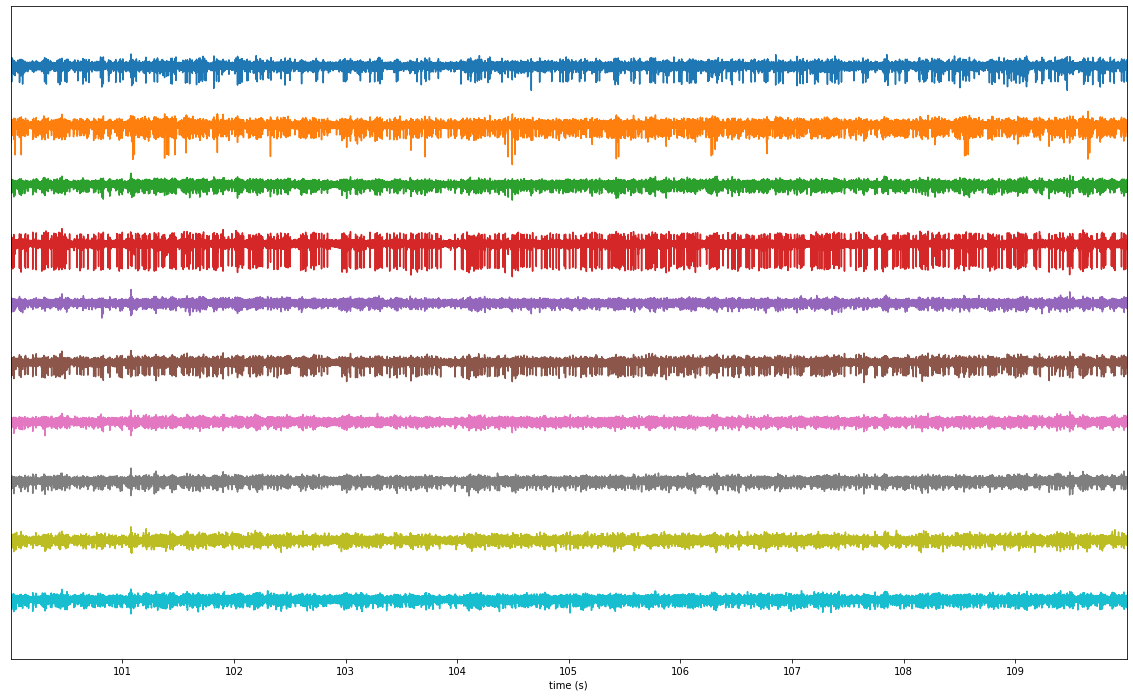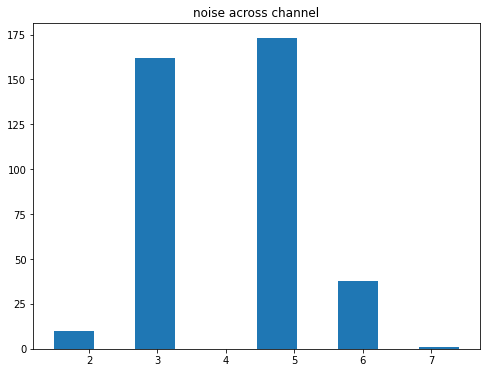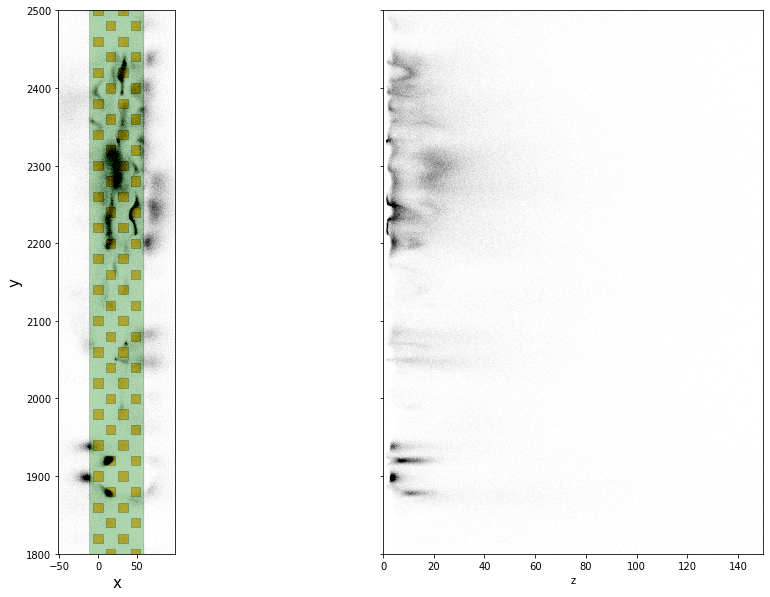# spikeinterface motion estimation / correction

## motion estimation in spikeinterface¶

In 2021,the SpikeInterface project has started to implemented `sortingcomponents`, a modular module for spike sorting steps.

Here is an overview or our progress integrating motion (aka drift) estimation and correction.

This notebook will be based on the open dataset from Nick Steinmetz published in 2021 "Imposed motion datasets" from Steinmetz et al. Science 2021 https://figshare.com/articles/dataset/_Imposed_motion_datasets_from_Steinmetz_et_al_Science_2021/14024495

The motion estimation is done in several modular steps:

1. detect peaks
2. localize peaks:
3. estimation motion:
• rigid or non rigid
• "decentralize" by Erdem Varol and Julien Boussard DOI : 10.1109/ICASSP39728.2021.9414145
• "motion cloud" by Julien Boussard (not implemented yet)

Here we will show this chain:

• detect peak > localize peaks with "monopolar_triangulation" > estimation motion "decentralize"
In :
```%load_ext autoreload
```
In :
```from pathlib import Path

import spikeinterface.full as si

import numpy as np
import matplotlib.pyplot as plt
plt.rcParams["figure.figsize"] = (20, 12)

from probeinterface.plotting import plot_probe
```
```/home/samuel.garcia/.virtualenvs/py38/lib/python3.8/site-packages/datalad/cmd.py:375: RuntimeWarning: coroutine 'run_async_cmd' was never awaited
new_loop = True
RuntimeWarning: Enable tracemalloc to get the object allocation traceback
/home/samuel.garcia/Documents/SpikeInterface/spikeinterface/spikeinterface/core/datasets.py:12: RuntimeWarning: coroutine 'run_async_cmd' was never awaited
RuntimeWarning: Enable tracemalloc to get the object allocation traceback
```
In :
```# local folder
base_folder = Path('/mnt/data/sam/DataSpikeSorting/imposed_motion_nick')

dataset_folder = base_folder / 'dataset1/NP1'
preprocess_folder = base_folder / 'dataset1_NP1_preprocessed'
peak_folder = base_folder / 'dataset1_NP1_peaks'

peak_folder.mkdir(exist_ok=True)
```
In :
```# global kwargs for parallel computing
job_kwargs = dict(
n_jobs=40,
chunk_memory='10M',
progress_bar=True,
)
```
In :
```# read the file
rec
```
Out:
`SpikeGLXRecordingExtractor: 384 channels - 1 segments - 30.0kHz - 1957.191s`
In :
```fig, ax = plt.subplots()
si.plot_probe_map(rec, ax=ax)
ax.set_ylim(-150, 200)
```
Out:
`(-150.0, 200.0)`### preprocess¶

This take 4 min for 30min of signals

In :
```if not preprocess_folder.exists():
rec_filtered = si.bandpass_filter(rec, freq_min=300., freq_max=6000.)
rec_preprocessed = si.common_reference(rec_filtered, reference='global', operator='median')
rec_preprocessed.save(folder=preprocess_folder, **job_kwargs)
```
In :
```# plot and check spikes
si.plot_timeseries(rec_preprocessed, time_range=(100, 110), channel_ids=rec.channel_ids[50:60])
```
Out:
`<spikeinterface.widgets.timeseries.TimeseriesWidget at 0x7f72adda0520>`### estimate noise¶

In :
```noise_levels = si.get_noise_levels(rec_preprocessed, return_scaled=False)
fig, ax = plt.subplots(figsize=(8,6))
ax.hist(noise_levels, bins=10)
ax.set_title('noise across channel')
```
Out:
`Text(0.5, 1.0, 'noise across channel')`### detect peaks¶

This take 1min30s

In :
```from spikeinterface.sortingcomponents.peak_detection import detect_peaks
```
In :
```if not (peak_folder / 'peaks.npy').exists():
peaks = detect_peaks(
rec_preprocessed,
method='locally_exclusive',
peak_sign='neg',
detect_threshold=5,
n_shifts=5,
noise_levels=noise_levels,
**job_kwargs,
)
np.save(peak_folder / 'peaks.npy', peaks)
print(peaks.shape)
```
```(4041179,)
```

### localize peaks¶

Here we chosse 'monopolar_triangulation' with log barrier

In :
```from spikeinterface.sortingcomponents.peak_localization import localize_peaks
```
In :
```if not (peak_folder / 'peak_locations_monopolar_triangulation_log_limit.npy').exists():
peak_locations = localize_peaks(
rec_preprocessed,
peaks,
ms_before=0.3,
ms_after=0.6,
method='monopolar_triangulation',
method_kwargs={'local_radius_um': 100., 'max_distance_um': 1000., 'optimizer': 'minimize_with_log_penality'},
**job_kwargs,
)
np.save(peak_folder / 'peak_locations_monopolar_triangulation_log_limit.npy', peak_locations)
print(peak_locations.shape)
```
In :
```print(peak_locations)
```
```[( 18.81849235, 1782.84538913,  78.17532357, 1696.96239445)
( 31.90279769, 3847.75061369, 134.79844077, 1716.03155721)
(-23.12038001, 2632.87834759,  87.76916268, 2633.62546695) ...
( 40.0839554 , 1977.83852796,  26.50998809, 1092.53885299)
(-51.40036701, 1772.34521905, 170.65660676, 2533.03617278)
( 54.3813594 , 1182.28971165,  87.35020554, 1303.53392431)]
```

### plot on probe¶

In :
```fig, axs = plt.subplots(ncols=2, sharey=True, figsize=(15, 10))
ax = axs
si.plot_probe_map(rec_preprocessed, ax=ax)
ax.scatter(peak_locations['x'], peak_locations['y'], color='k', s=1, alpha=0.002)
ax.set_xlabel('x')
ax.set_ylabel('y')
if 'z' in peak_locations.dtype.fields:
ax = axs
ax.scatter(peak_locations['z'], peak_locations['y'], color='k', s=1, alpha=0.002)
ax.set_xlabel('z')
ax.set_xlim(0, 150)
ax.set_ylim(1800, 2500)
```
Out:
`(1800.0, 2500.0)`### plot peak depth vs time¶

In :
```fig, ax = plt.subplots()
x = peaks['sample_ind'] / rec_preprocessed.get_sampling_frequency()
y = peak_locations['y']
ax.scatter(x, y, s=1, color='k', alpha=0.05)
ax.set_ylim(1300, 2500)
```
Out:
`(1300.0, 2500.0)`